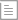资讯

# AE入门教程，5个最常用的AE表达式详解

2017-12-05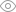2073 分类：设计资讯

AE入门教程，5个最常用的AE表达式详解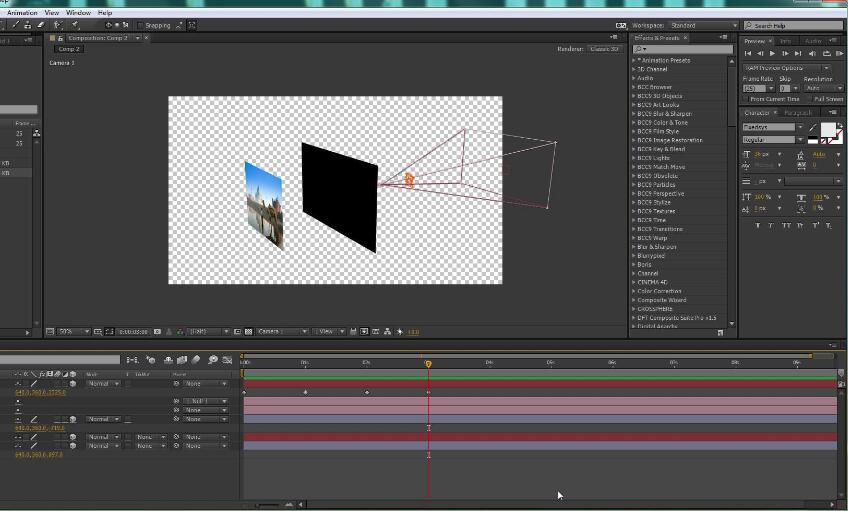1.弹性表达式 Bounce

n = 0;

if (numKeys > 0){

n = nearestKey(time).index;

if (key(n).time > time){

n--;

}

}

if (n == 0){

t = 0;

}else{

t = time - key(n).time;

}

if (n > 0 && t < 1){

v = velocityAtTime(key(n).time - thisComp.frameDuration/10);

amp = .06;

freq = 3;

decay = 5.0;

value + v*amp*Math.sin(freq*t*2*Math.PI)/Math.exp(decay*t);

}else{

value;

}

2.抖动表达式 wiggle

wiggle就不用多说了吧，很常用，代码为 wiggle（x，y），第一个参数X表示抖动频率 即1秒抖动多少次，第二个参数表示抖动幅度 具体需要根据你视频片段确定参数。

3. 挤压与伸展 效果类似于果冻

maxDev = 13; // max deviation in pixels

spd = 30; //speed of oscillation

decay = 1.0; //how fast it slows down

t = time - inPoint;

x = scale + maxDev*Math.sin(spd*t)/Math.exp(decay*t);

y = scale*scale/x;

[x,y]

4. 运动拖尾

delay = 5; //number of frames to delay

d = delay*thisComp.frameDuration*(index - 1);

thisComp.layer(1).position.valueAtTime(time - d)

opacityFactor = .75;

Math.pow(opacityFactor,index - 1)*100

5. 计时&倒计时

//Define time values

var hour = Math.floor((time/60)/60);

var min = Math.floor(time/60);

var sec = Math.floor(time);

var mili = Math.floor(time*60);

// Cleaning up the values

if (mili > 59){ mili = mili - sec*60; }

if (mili < 10){ mili = "0" + mili; } if (sec > 59){ sec = sec - min*60; }

if (sec < 10){ sec = "0" + sec; } if (min >= 59){ min = min - hour*60; }

if (min < 10){ min = "0" + min; }

// no hour cleanup

if (hour < 10){ hour = "0" + hour; }

//Output

hour + ' : ' + min + ' : ' + sec + ' : ' + mili;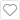收藏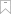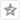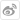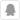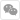Rhino

keyshot

Pro/Engineer

Photoshop

IF概念学生设计大赛

# 官方微信400-088-6822